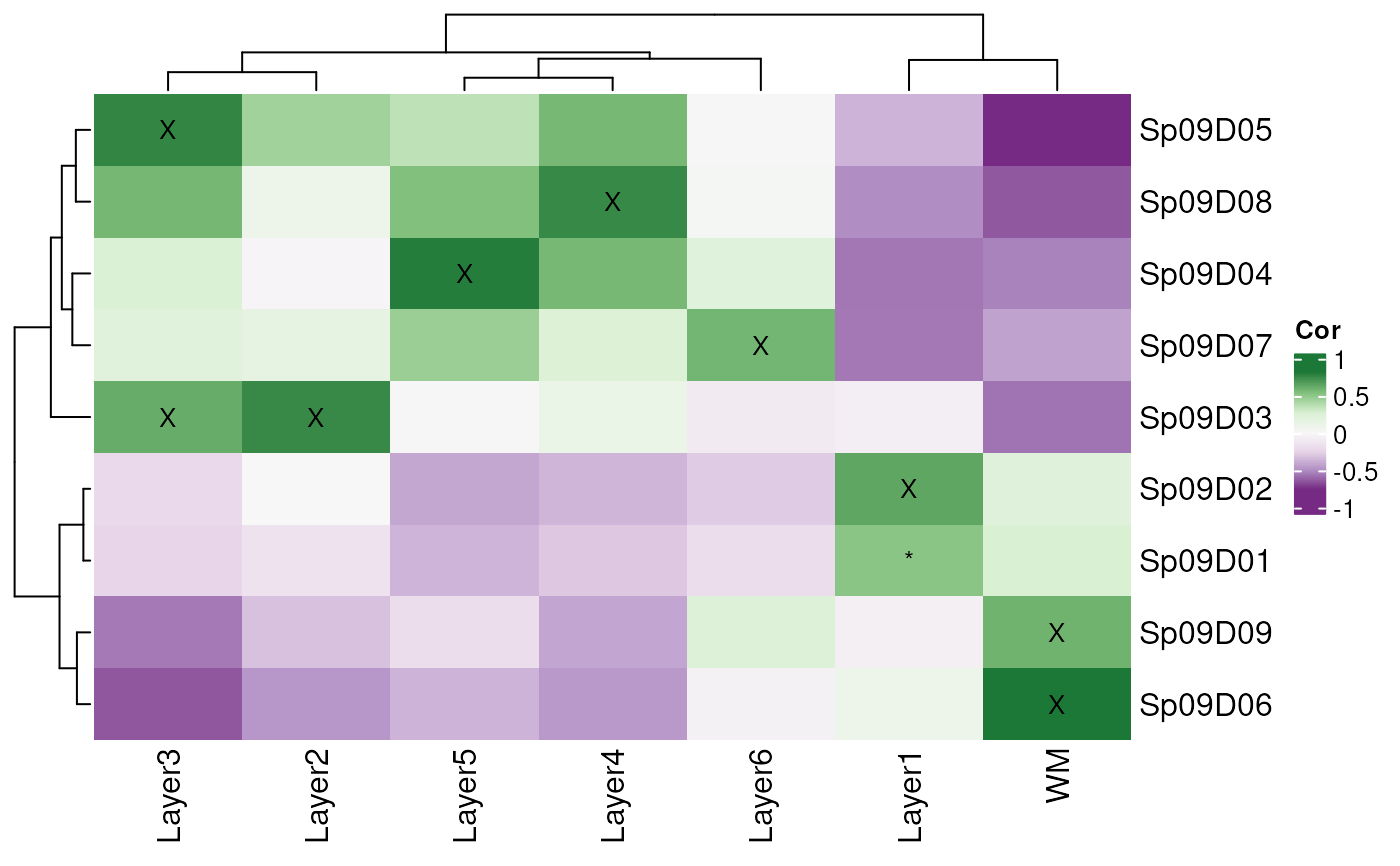This function makes a heatmap from the layer_stat_cor() correlation matrix between a given set of cell cluster/type statistics derived from scRNA-seq or snRNA-seq data (among other types) and the layer statistics from the Human DLPFC Visium data (when using the default arguments).

layer_stat_cor_plot(
cor_stats_layer,
max = 0.81,
min = -max,
layerHeights = NULL,
cex = 1.2
)

## Arguments

cor_stats_layer

The output of layer_stat_cor().

max

A numeric(1) specifying the highest correlation value for the color scale (should be between 0 and 1).

min

A numeric(1) specifying the lowest correlation value for the color scale (should be between 0 and -1).

layerHeights

A numeric() vector of length equal to ncol(cor_stats_layer) + 1 that starts at 0 specifying where to plot the y-axis breaks which can be used for re-creating the length of each brain layer. Gets passed to layer_matrix_plot().

cex

Passed to layer_matrix_plot().

## Value

A heatmap for the correlation matrix between statistics.

## Details

Check https://github.com/LieberInstitute/HumanPilot/blob/master/Analysis/Layer_Guesses/dlpfc_snRNAseq_annotation.R for a full analysis from which this family of functions is derived from.

layer_matrix_plot annotate_registered_clusters

Other Layer correlation functions: annotate_registered_clusters(), layer_stat_cor()

## Examples


## Obtain the necessary data
if (!exists("modeling_results")) {
modeling_results <- fetch_data(type = "modeling_results")
}

## Compute the correlations
cor_stats_layer <- layer_stat_cor(
tstats_Human_DLPFC_snRNAseq_Nguyen_topLayer,
modeling_results,
model_type = "enrichment"
)

## Visualize the correlation matrix
layer_stat_cor_plot(cor_stats_layer, max = max(cor_stats_layer))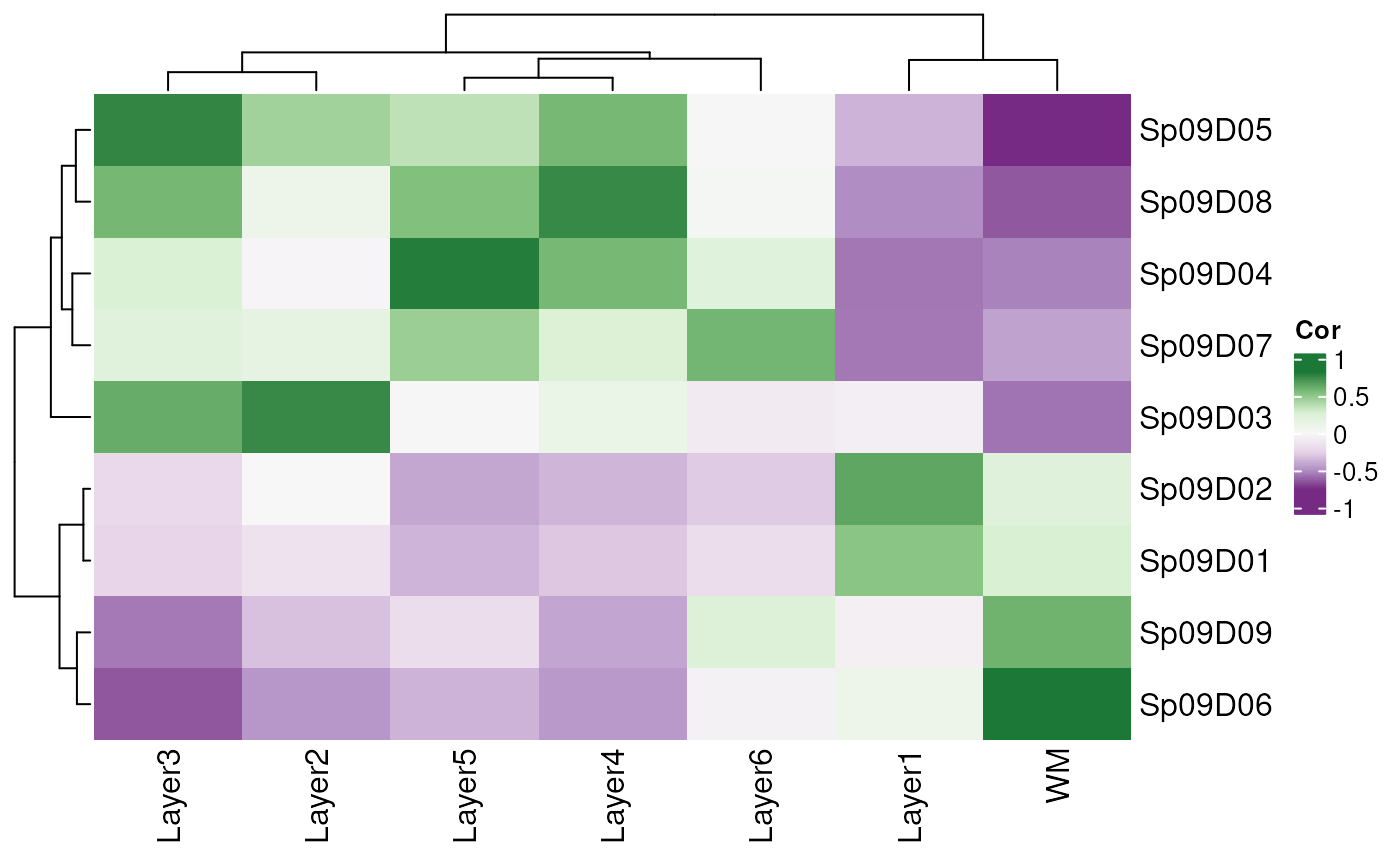## Annotate then re-plot
rownames(cor_stats_layer) <- paste0(
rownames(cor_stats_layer),
" - ",
annotate_registered_clusters(cor_stats_layer)\$layer_label
)
layer_stat_cor_plot(cor_stats_layer, max = max(cor_stats_layer))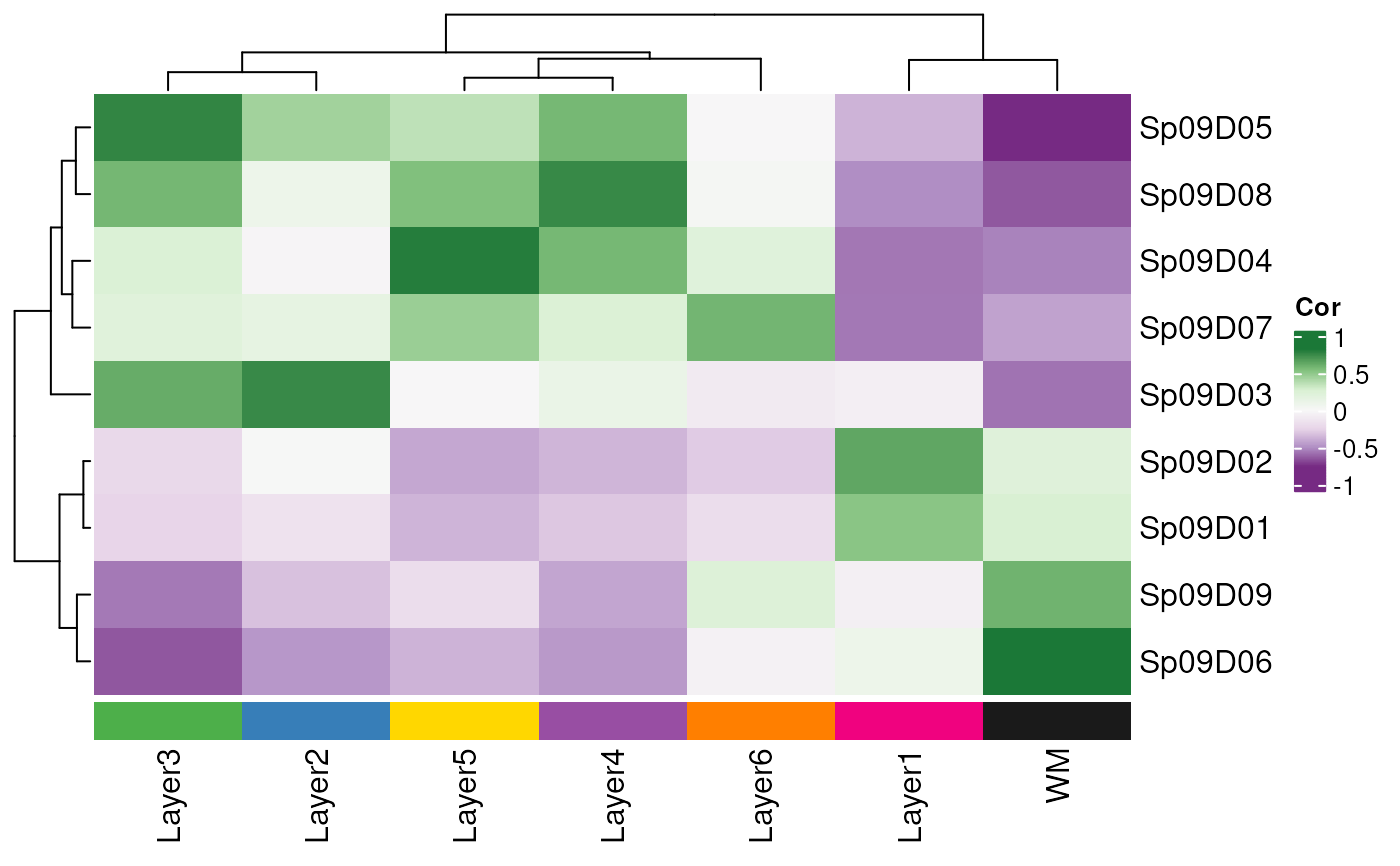## Restrict the range of colors further
layer_stat_cor_plot(cor_stats_layer, max = 0.25)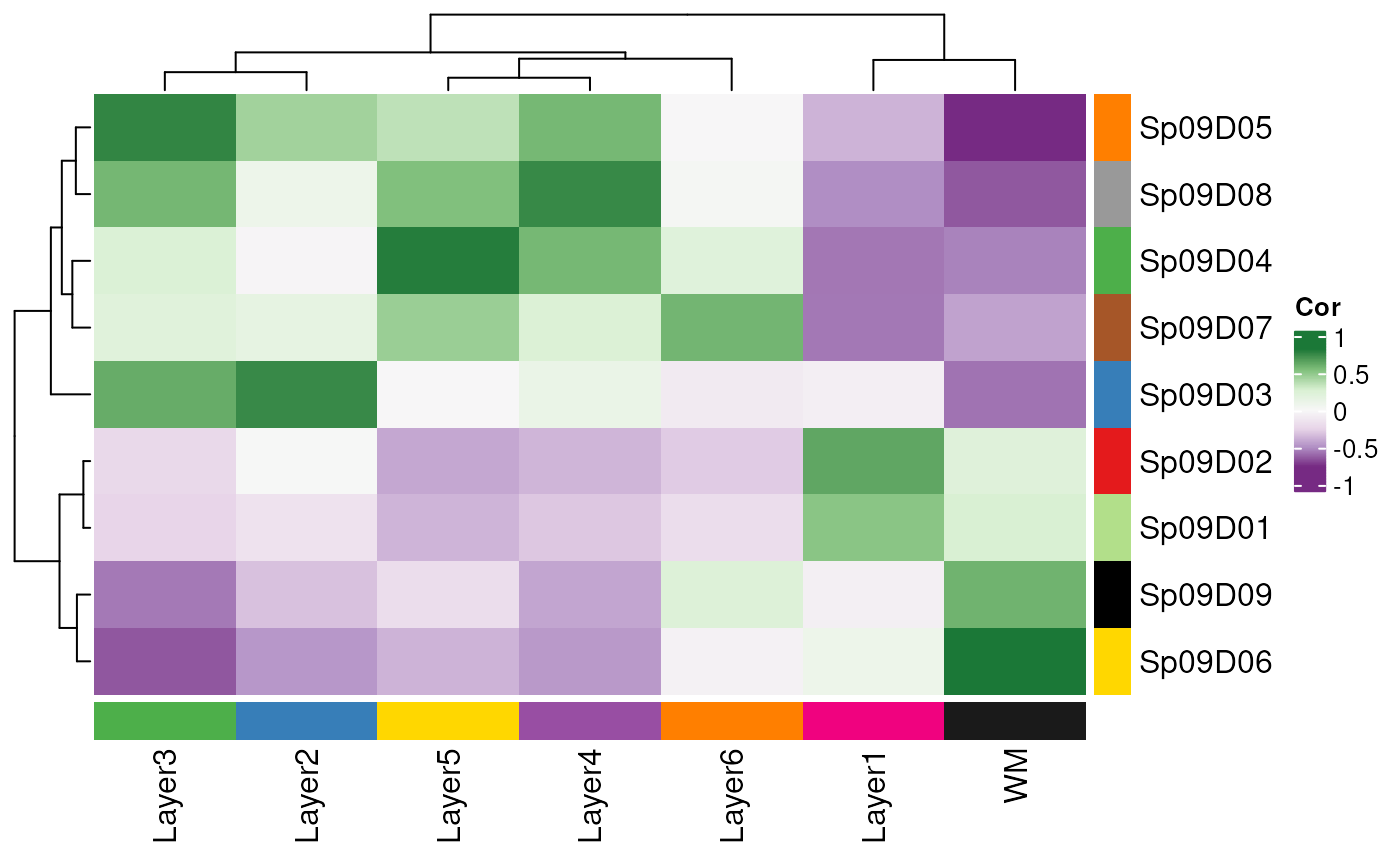## Repeat with just the top 10 layer marker genes
layer_stat_cor_plot(layer_stat_cor(
tstats_Human_DLPFC_snRNAseq_Nguyen_topLayer,
modeling_results,
model_type = "enrichment",
top_n = 10
), max = 0.25)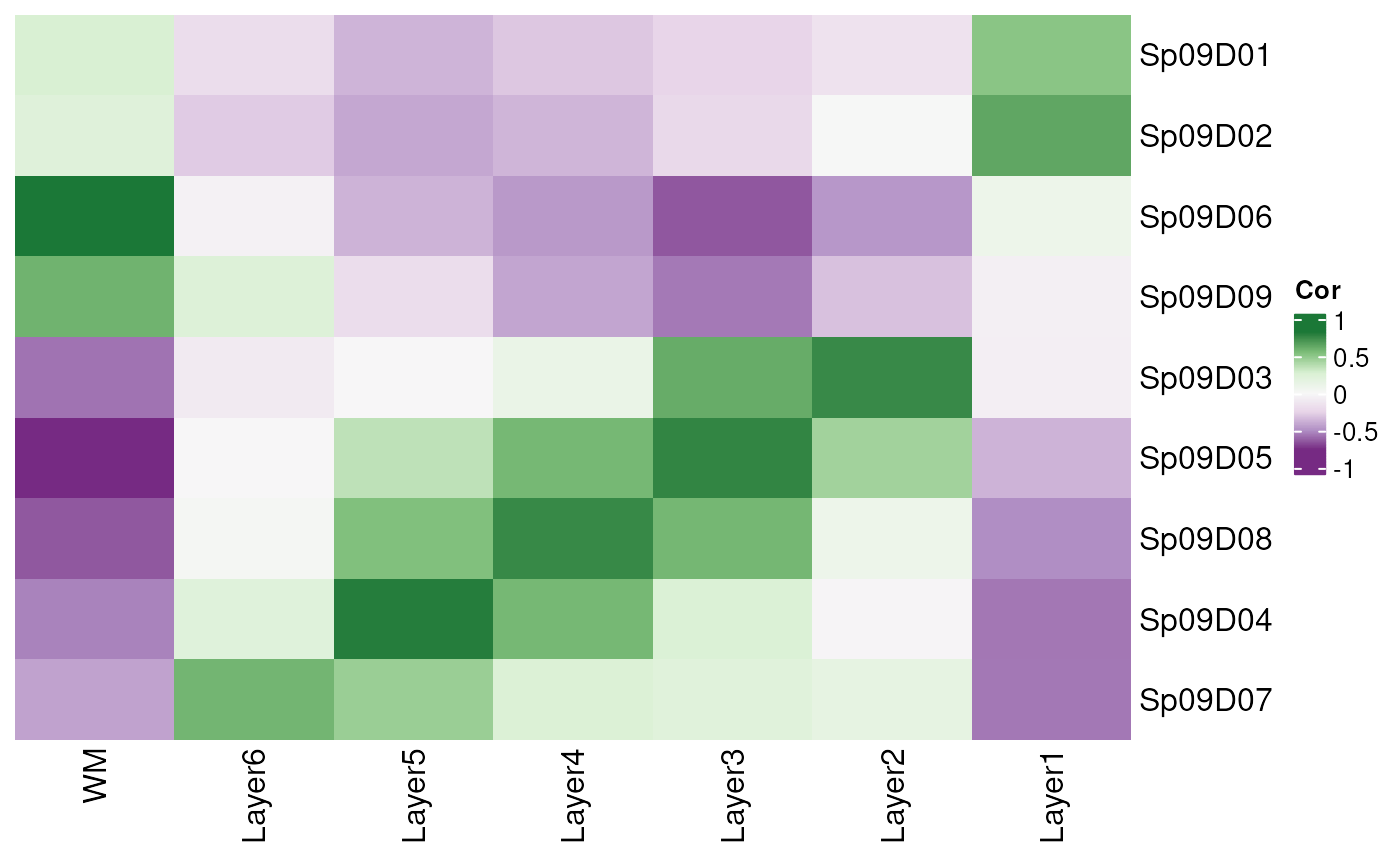## Now with the "pairwise" modeling results and also top_n = 10
layer_stat_cor_plot(layer_stat_cor(
tstats_Human_DLPFC_snRNAseq_Nguyen_topLayer,
modeling_results,
model_type = "pairwise",
top_n = 10
), max = 0.25)